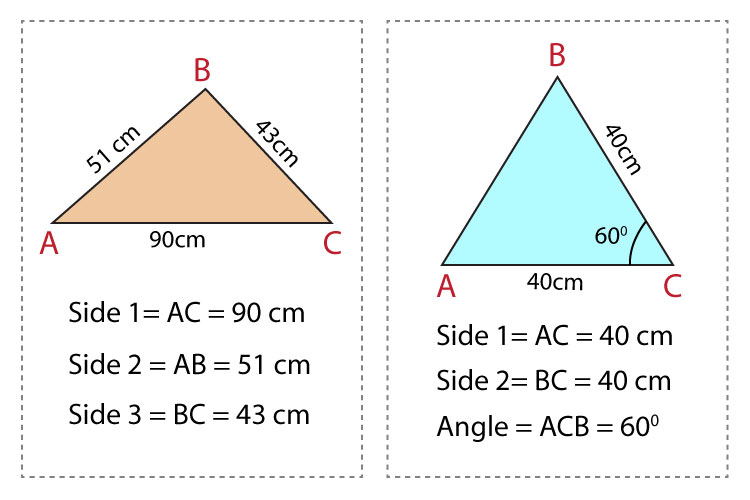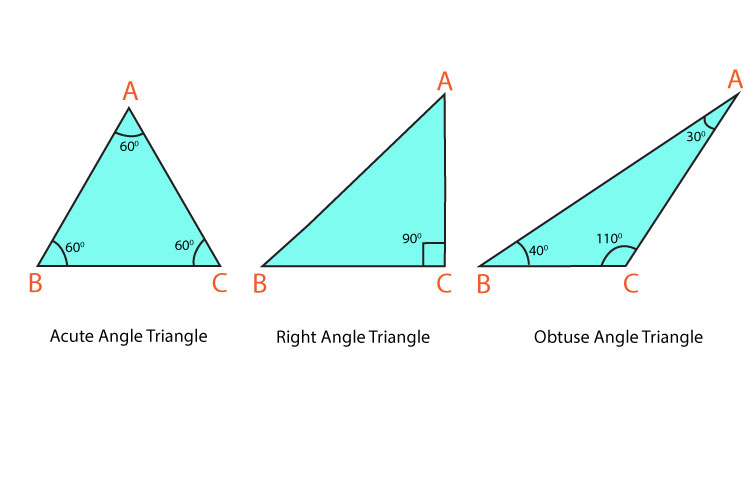# What is the cosine rule and when can it be used?

Cosine Rule is used:

When the length of all 3 sides of a triangle is given. OR
When 2 sides and an included angle is given. (C is an included angle of lines 43 cm and 90 cm.)Cosine is a trigonometric function used to calculate angles of a right-angled triangle. However, the cosine rule can be used for all types of triangles including acute angled, right-angled, and obtuse-angled triangles.Cosine Rule:
a.  To calculate the length of sides.

a2 = b2 + c2 - 2bc cos A
b2 = a2 + c2 - 2ac cos B
c2 = b2 + c2 - 2ab cos C

b.  To calculate the size of angles.

a2 = b2 + c2 - 2bc cos A
a2 + 2bc cos A = b2 + c2 - 2bc cos A + 2bc cos A (add 2bc cos A on both sides)
a2 - a2 + 2bc cos A = b2 + c2 - a2  (subtract a2 on both sides)
2bc cos A = b2 + c2 - a2    (divide 2bc on both sides)
cos A=  (b^2  + c^2  - a^(2 ))/2bc
cos A=  (b^2  + c^2  - a^(2 ))/2bc
cos B=  (a^2  + c^2  - b^(2 ))/2ac
cos C=  (a^2  + b^2  - c^(2 ))/2ac

1. Label the triangle
a.  Label the angles A, B and C.
Any angle can be labelled as A, B or C.
b.  Label the sides a, b, c.
The side opposite to angle A is labelled a, the side opposite to angle B is labelled
b, and the side opposite to angle C is labelled c.In the triangle, a = 43 cm, b = 90 cm, c = 51 cm.

To calculate angle A:

cos A=  (b2  + c2  - a2 /2bc

cos A=  (902  + 512  - 432 /(2*51*90)

cos A= 0.964

A= cos-1 (0.964)

A=15.420571°